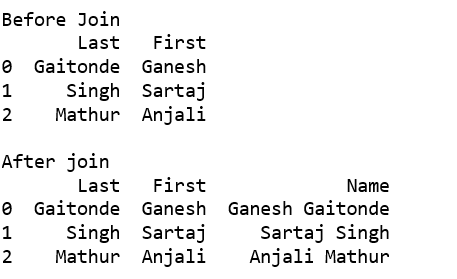# Join two text columns into a single column in Pandas

Let’s see the different methods to join two text columns into a single column.

Method #1: Using cat() function
We can also use different separators during join. e.g. -, _, ” ” etc.

 `# importing pandas ` `import` `pandas as pd ` ` `  `df ``=` `pd.DataFrame({``'Last'``: [``'Gaitonde'``, ``'Singh'``, ``'Mathur'``], ` `                   ``'First'``: [``'Ganesh'``, ``'Sartaj'``, ``'Anjali'``]}) ` ` `  `print``(``'Before Join'``) ` `print``(df, ``'\n'``) ` ` `  `print``(``'After join'``) ` `df[``'Name'``] ``=` `df[``'First'``].``str``.cat(df[``'Last'``], sep ``=``" "``) ` `print``(df) `

Output :Method #2: Using lambda function

This method generalizes to an arbitrary number of string columns by replacing df[[‘First’, ‘Last’]] with any column slice of your dataframe, e.g. df.iloc[:, 0:2].apply(lambda x: ‘ ‘.join(x), axis=1).

 `# importing pandas ` `import` `pandas as pd ` ` `  `df ``=` `pd.DataFrame({``'Last'``: [``'Gaitonde'``, ``'Singh'``, ``'Mathur'``], ` `                   ``'First'``: [``'Ganesh'``, ``'Sartaj'``, ``'Anjali'``]}) ` ` `  `print``(``'Before Join'``) ` `print``(df, ``'\n'``) ` ` `  `print``(``'After join'``) ` `df[``'Name'``] ``=` `df[[``'First'``, ``'Last'``]].``apply``(``lambda` `x: ``' '``.join(x), axis ``=` `1``) ` `print``(df) `

Output :Method #3: Using + operator

We need to convert data frame elements into string before join. We can also use different separators during join, e.g. -, _, ‘ ‘ etc.

 `# importing pandas ` `import` `pandas as pd ` ` `  `df ``=` `pd.DataFrame({``'Last'``: [``'Gaitonde'``, ``'Singh'``, ``'Mathur'``], ` `                   ``'First'``: [``'Ganesh'``, ``'Sartaj'``, ``'Anjali'``]}) ` ` `  `print``(``'Before Join'``) ` `print``(df, ``'\n'``) ` ` `  `print``(``'After join'``) ` `df[``'Name'``]``=` `df[``"First"``].astype(``str``) ``+``" "``+` `df[``"Last"``] ` `print``(df) `

Output :Whether you're preparing for your first job interview or aiming to upskill in this ever-evolving tech landscape, GeeksforGeeks Courses are your key to success. We provide top-quality content at affordable prices, all geared towards accelerating your growth in a time-bound manner. Join the millions we've already empowered, and we're here to do the same for you. Don't miss out - check it out now!

Previous
Next